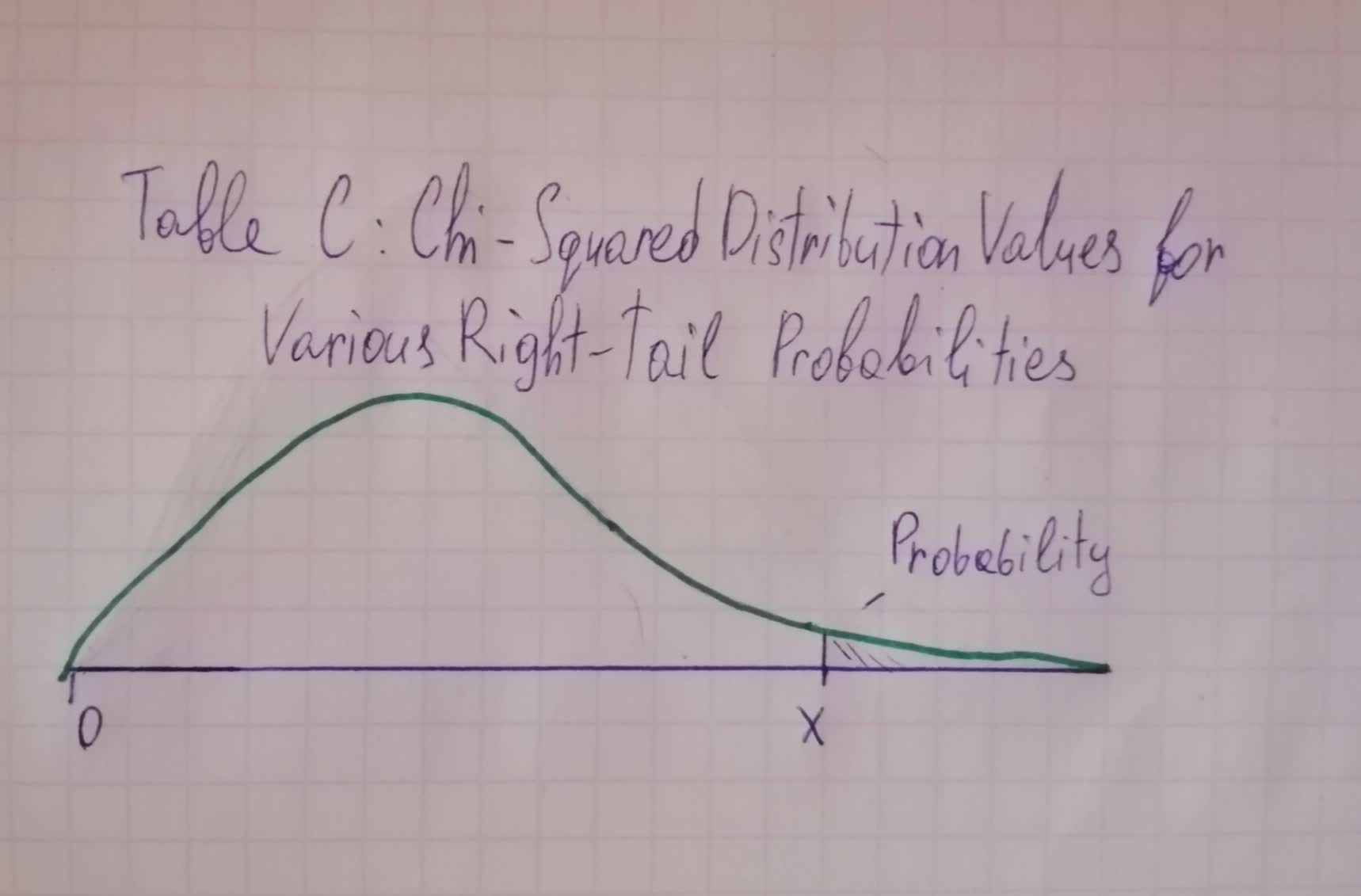Get help with statistics and probability

Recent questions in Statistics and Probabilitydanrussekme 2021-11-08 Answered

The random variables X and Y have joint density function f(x,y)=12xy(1-x) 0 and equal to 0 otherwise. Find E[X].Minerva Kline 2021-11-08 Answered

If X is a normal random variable with parameters $$\displaystyle\mu={10}$$ and $$\displaystyle\sigma^{{{2}}}={36}$$ , compute P[X>16]Pearl Carney 2021-11-08 Answered

If X is a normal random variable with parameters $$\displaystyle\mu={10}$$ and $$\displaystyle\sigma^{{{2}}}={36}$$ , compute P[X<8]chanyingsauu7 2021-11-07 Answered

Let X be a normal random variable with mean 12 and variance 4. Find the value of c such that P[X>c]=.10.York 2021-11-07 Answered

Random variables X and Y have joint PDF $$f_{X,Y}(x,y)=\begin{cases}12e^{-(3x+4y)},\ x \geq 0, y \geq 0\\0,\ otherwise\end{cases}$$ Find $$\displaystyle{P}{\left[{X}+{Y}\leq{1}\right]}$$verskalksv 2021-11-06 Answered

If X is a normal random variable with parameters $$\displaystyle\mu={10}$$ and $$\displaystyle\sigma^{{{2}}}={36}$$ , compute P[X>5]Emeli Hagan 2021-11-06 Answered

Which of the following are assumptions for the Significance Test for the Proportion? 1) Data is Categorical. 2) Data is from a convenience sample. 3) $$\displaystyle{n}{p}_{{{0}}}$$ and $$\displaystyle{\left({1}-{p}_{{{0}}}\right)}$$ are both greater than 15 4) Data is Quantitative 5) Data is from a Normal Distribution 6) Data is from a random sample 7) $$\displaystyle\hat{{{n}{p}}}$$ and $$\displaystyle{n}{\left({1}-\hat{{{p}}}\right)}$$ are both greater than 15alesterp 2021-11-06 Answered

Using the Standard Normal Table from the online lectures this week, what is the area under the standard normal curve: a) To the left of a z-score of 1.25 b) To the right of a z-score of 1.25 c) Between the z-scores -0.25 and 0.55EunoR 2021-11-06 Answered

Let $$\displaystyle{X}_{{{1}}}\ldots.,{X}_{{{n}}}{\quad\text{and}\quad}{Y}_{{{1}}},\ldots,{Y}_{{{m}}}$$ be two sets of random variables. Let $$\displaystyle{a}_{{{i}}},{b}_{{{j}}}$$ be arbitrary constant. Show that $$\displaystyle{C}{o}{v}{\left({\sum_{{{i}={1}}}^{{{n}}}}{a}_{{{i}}}{X}_{{{i}}},{\sum_{{{j}={1}}}^{{{m}}}}{b}_{{{j}}}{Y}_{{{j}}}\right)}={\sum_{{{i}={1}}}^{{{n}}}}{\sum_{{{j}={1}}}^{{{m}}}}{a}_{{{i}}}{b}_{{{j}}}{C}{o}{v}{\left({X}_{{{i}}},{Y}_{{{j}}}\right)}$$tinfoQ 2021-11-05 Answered

$\begin{array}{cc}\hline & \text{Afraid to walk at night?} \\ \hline & \text{Yes} & \text{No} & \text{Total} \\ \hline \text{Male} & 173 & 598 & 771 \\ \hline \text{Female} & 393 & 540 & 933 \\ \hline \text{Total} & 566 & 1138 & 1704 \\ \hline \text{Source:}2014\ GSS \end{array}$ If the chi-square $$\displaystyle{\left(\chi{2}\right)}$$ test statistic $$\displaystyle={73.7}$$ what is the p-value you would report? Use Table C.Remember to calculate the df first. Group of answer choices $$\displaystyle{P}{>}{0.250}$$ $$\displaystyle{P}={0.01}$$ $$\displaystyle{P}{<}{0.001}$$ $$\displaystyle{P}{<}{0.002}$$$\begin{array}{|c|cc|}\hline & \text{Right-Tail Probability} \\ \hline df & 0.250 & 0.100 & 0.050 & 0.025 & 0.010 & 0.005 & 0.001 \\ \hline 1 & 1.32 & 2.71 & 3.84 & 5.02 & 6.63 & 7.88 & 10.83 \\ 2 & 2.77 & 4.61 & 5.99 & 7.38 & 9.21 & 10.60 & 13.82 \\ 3 & 4.11 & 6.25 & 7.81 & 9.35 & 11.34 & 12.84 & 16.27 \\ 4 & 5.39 & 7.78 & 9.49 & 11.14 & 13.28 & 14.86 & 18.47 \\ 5 & 6.63 & 9.24 & 11.07 & 12.83 & 15.09 & 16.75 & 20.52 \\ 6&7.84&10.64&12.59&14.45&16.81&18.55&22.46 \\ 7&9.04&12.02&14.07&16.01&18.48&20.28&24.32\\ 8&10.22&13.36&15.51&17.53&20.09&21.96&26.12 \\ 9&11.39&14.68&16.92&19.02&21.67&23.59&27.88 \\ 10&12.55&15.99&18.31&20.48&23.21&25.19&29.59 \\ 11&13.70&17.28&19.68&21.92&24.72&26.76&31.26 \\ 12&14.85&18.55&21.03&23.34&26.22&28.30&32.91 \\ 13&15.98&19.81&22.36 & 24.74 & 27.69 & 29.82 & 34.53 \\ 14 & 17.12 & 21.06 & 23.68 & 26.12 & 29.14 & 31.32 & 36.12 \\15 & 18.25 & 22.31 & 25.00 & 27.49 & 30.58 & 32.80 & 37.70 \\ 16 & 19.37 & 32.54 & 26.30 & 28.85 & 32.00 & 34.27 & 39.25 \\ 17 & 20.49 & 24.77 & 27.59 & 30.19 & 33.41 & 35.72 & 40.79 \\ 18 & 21.60 & 25.99 & 28.87 & 31.53 & 34.81 & 37.16 & 42.31 \\ 19 & 22.72 & 27.20 & 30.14 & 32.85 & 36.19 & 38.58 & 43.82 \\ 20 & 23.83 & 28.41 & 31.41 & 34.17 & 37.57 & 40.00 & 45.32 \\ \hline \end{array}$Ramsey 2021-11-05 Answered

Determine whether the statement is true or false. If it is false, rewrite it as a true statement: "when each data class has the same frequency, the distribution is symmetric."avissidep 2021-11-05 Answered

For a test of $$\displaystyle{H}_{{{0}}}:\ {p}={0.5},$$ the z test statistic equals 1.74. Find the p-value for $$\displaystyle{H}_{{{a}}}:\ {p}{>}{0.5}$$. a) 0.0446 b) 0.0409 c) 0.892 d) 0.9591 e) 0.0818 f) 0.9554sibuzwaW 2021-11-05 Answered

Random variables X and Y have joint PDF $$f_{X,Y}(x,y)=\begin{cases}12e^{-(3x+4y)},\ x \geq 0, y \geq 0\\0,\ otherwise\end{cases}$$ Find $$\displaystyle{P}{\left[\min{\left({X},{Y}\right)}\geq{2}\right]}$$Kye 2021-11-05 Answered

The joint density of the random variables X and Y is given by $$f(x,y)=\begin{cases}8xy & 0\leq x\leq 1, 0\leq y \leq x\\0 & otherwise\end{cases}$$ Find the marginal density of XBrennan Flores 2021-11-04 Answered

Compute the distribution of X+Y in the following cases: X and Y are independent normal random variables with respective parameters $$\displaystyle{\left(\mu_{{{1}}},{\sigma_{{{1}}}^{{{2}}}}\right)}{\quad\text{and}\quad}{\left(\mu_{{{2}}},{\sigma_{{{2}}}^{{{2}}}}\right)}$$.Dolly Robinson 2021-11-03 Answered

For continuous random variables X and Y with joint probability density function $$f(x,y)=\begin{cases}xe^{-(x+xy)} & x>0\ and\ y>0\\0 & otherwise\end{cases}$$ Find P(X>1 and Y>1).Trent Carpenter 2021-11-03 Answered

The joint density of the random variables X and Y is given by $$f(x,y)=\begin{cases}8xy & 0\leq x\leq 1, 0\leq y \leq x\\0 & otherwise\end{cases}$$ Find the conditional density of X Find the conditional density of YSuman Cole 2021-11-01 Answered

Two random variables X and Y with joint density function given by: $$f(x,y)=\begin{cases}\frac{1}{3}(2x+4y)& 0\leq x,\leq 1\\0 & elsewhere\end{cases}$$ Find the marginal density of X.sanuluy 2021-11-01 Answered

Assume that X and Y are jointly continuous random variables with joint probability density function given by $$\displaystyle{f{{\left({x},{y}\right)}}}={b}{e}{g}\in{\left\lbrace{c}{a}{s}{e}{s}\right\rbrace}{\frac{{{1}}}{{{36}}}}{\left({3}{x}-{x}{y}+{4}{y}\right)}\ {\quad\text{if}\quad}\ {0}{<}{x}{<}{2}\ {\quad\text{and}\quad}\ {1}{<}{y}{<}{3}\backslash{0}\ \ \ \ \ {o}{t}{h}{r}{e}{w}{i}{s}{e}{e}{n}{d}{\left\lbrace{c}{a}{s}{e}{s}\right\rbrace}$$ Find the marginal density functions for X and Y .Efan Halliday 2021-10-30 Answered

Compute the distribution of X+Y in the following cases: X and Y are independent Poisson random variables with means respective $$\displaystyle\lambda_{{{1}}}{\quad\text{and}\quad}\lambda_{{{2}}}$$.

Regardless of what statistical task you might be approaching, examples of quantitative data must be explored first to determine the objectives.
...